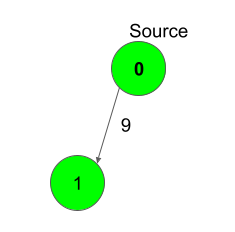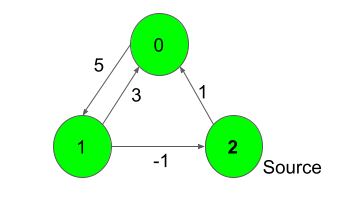XDAYS

:

HOUR

:

MINS

:

SEC

Copied to Clipboard
Distance from the Source (Bellman-Ford Algorithm)
Medium Accuracy: 56.6% Submissions: 5036 Points: 4

Given a weighted, directed and connected graph of V vertices and E edges, Find the shortest distance of all the vertex's from the source vertex S.
Note: The Graph doesn't contain any negative weight cycle.

Example 1:

Input:S = 0
Output:
0 9
Explanation:
Shortest distance of all nodes from
source is printed.

Example 2:

Input:S = 2
Output:
1 6 0
Explanation:
For nodes 2 to 0, we can follow the path-
2-0. This has a distance of 1.
For nodes 2 to 1, we cam follow the path-
2-0-1, which has a distance of 1+5 = 6,

You don't need to read input or print anything. Your task is to complete the function bellman_ford()
which takes number of vertices V and an E sized list of lists of three integers where the three integers are u,v, and w; denoting there's an edge from u to v, which has a weight of w as input parameters and returns a list of integers where the ith integer denotes the distance of ith node from source node. If some node isn't possible to visit, then it's distance should be 100000000(1e8).

Expected Time Complexity: O(V*E).
Expected Auxiliary Space: O(V).

Constraints:
1
V 500
1
E V*(V-1)
-1000
0
S < V

We are replacing the old Disqus forum with the new Discussions section given below.

Editorial

We strongly recommend solving this problem on your own before viewing its editorial. Do you still want to view the editorial?

My Submissions: